×#### Thank you for registering.

One of our academic counsellors will contact you within 1 working day.

Click to Chat

1800-1023-196

+91-120-4616500

CART 0

• 0

MY CART (5)

Use Coupon: CART20 and get 20% off on all online Study Material

ITEM
DETAILS
MRP
DISCOUNT
FINAL PRICE
Total Price: Rs.

There are no items in this cart.
Continue Shopping• Complete JEE Main/Advanced Course and Test Series
• OFFERED PRICE: Rs. 15,900
• View Details

```Chapter 3: Pair of Linear Equations in Two Variables Exercise – 3.11

Question: 1

If in a rectangle, the length is increased and breadth reduced each by 2 units, the area is reduced by 28 square units. If, however, the length is reduced by 1 unit and the breadth is increased by 2 units, the area increases by 33 square units. Find the area of the rectangle.

Solution:

Let the length and breadth of the rectangle be x and y units respectively.

Then, area of rectangle = xy units

If length is increased and breadth is reduced each by 2 units, then the area is reduced by 28 square units.

(x + 2)(y - 2) = xy - 28

⇒ xy − 2x + 2y − 4 = xy - 28

⇒ −2x + 2y − 4 + 28 = 0

⇒ −2x + 2y + 24 = 0

⇒ 2x − 2y − 24 = 0

Therefore, 2x – 2y – 24 = 0 …. (i)

Then the length is reduced by 1 unit and the breadth is increased by 2 units then the area is increased by 33 square units.

(x − 1)(y + 2) = xy + 33

⇒ xy + 2x − y − 2 = x + 33

⇒ 2x − y − 2 − 33 = 0

⇒ 2x − y − 35 = 0

Therefore, 2x - y - 35 = 0 ….. (ii)

Thus we get the following system of linear equation

2x - 2y - 24 = 0

2x - y - 35 = 0

By using cross multiplication, we have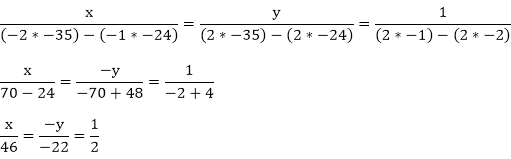x = 46/2

x = 23

And

y = 22/2

y = 11

The length of rectangle is 23 units.

The breadth of rectangle is 11 units.

Area of rectangle = length x breadth,

= x × y

= 23 × 11

= 253 square units

Hence, the area of rectangle is 253 square units.

Question: 2

The area of a rectangle remains the same if the length is increased by 7 metres and the breadth is decreased by 3 metres. The area remains unaffected if the length is decreased by 7 metres and the breadth is increased by 5 metres. Find the dimensions of the rectangle.

Solution:

Let the length and breadth of the rectangle be x and y units respectively

Then, area of rectangle = xy square units

If length is increased by 7 metres and breadth is decreased by 3 metres the area of a rectangle remains the same

Therefore,

xy = (x + 7)(y − 3)

xy = xy + 7y − 3x − 21

3x – 7y + 21 = 0 …. (i)

If the length is decreased by 7 metres and the breadth is increased by 5 metres the area remains unaffected, then

xy = (x − 7)(y + 5)

xy = xy − 7y + 5x − 35

5x – 7y – 35 = 0 …..(ii)

Thus we get the following system of linear equation

3x – 7y + 21 = 0

5x – 7y – 35 = 0

By using cross-multiplication, we have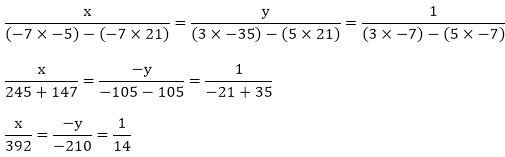x = 392/14

x = 28

AndHence, the length of rectangle is 28 meters

The breadth of rectangle is 15 metres

Question: 3

In a rectangle, if the length is increased by 3 metres and breadth is decreased by 4 metres, the area of the triangle is reduced by 67 square metres. If length is reduced by 1 metre and breadth is increased by 4 metres, the area is increased by 89 sq. metres. Find the dimension of the rectangle.

Solution:

Let the length and breadth of the rectangle be x and y units respectively

Then, area of rectangle = xy square units

If the length is increased by 3 metres and breadth is reduced by 4 metres, then the area is reduced by 67 sq. metres

Therefore,

xy – 67 = (x + 3)(y – 4)

xy – 67 = xy + 3y – 4x – 12

4xy – 3y – 67 + 12 = 0

4x – 3y – 55 = 0 — (i)

Then the length is reduced by 1 metre and the breadth is increased by 4 metre, then the area is increased by 89 sq. metres

Therefore,

4x – y – 93 = 0  ….. (ii)

Thus, we get the following system of linear equation

4x – 3y – 55 = 0

4x – y – 93 = 0

By using cross multiplication, we have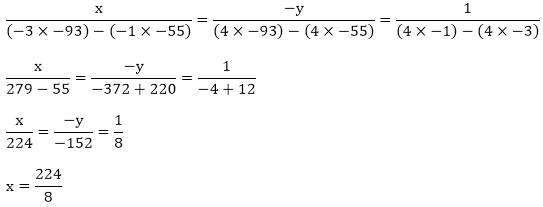x = 28

And

152/8

y = 19

Hence, the length of rectangle is 28 metre

The breadth of rectangle is 19 metre.

Question: 4

The income of X and Y are in the ratio of 8:7 and their expenditures are in the ratio 19:16. If each saves Rs. 1250, find their incomes.

Solution:

Let the income of X be Rs. 8x and the income of Y be Rs. 7x. Further let the expenditure of X be 19y and the expenditure of Y be 16y. Then,

Saving of X = 8x – 19y = 1250

Saving of Y = 7x – 16y = 1250

8x – 19y – 1250 = 0 …. (i)

7x – 16y – 1250 = 0 …. (ii)

Solving equation (i) and (ii) by cross-multiplication, we have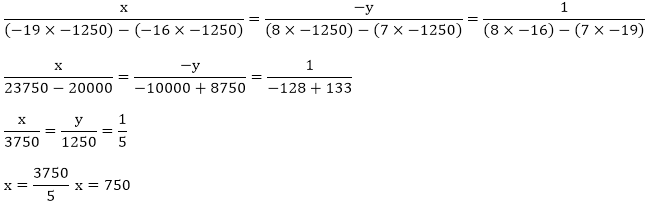The monthly income of X = 8x

= 8 × 750

= 6000

The monthly income of Y = 7x

= 7 × 750

= 5250

Hence the monthly income of X is Rs. 6000

The monthly income of Y is Rs. 5250

Question: 5

A and B each has some money. If A gives Rs. 30 to B, then B will have twice the money left with A. But, if B gives Rs. 10 to A, then A will have thrice as much as is left with B. How much money does each have?

Solution:

Let the money with A be Rs. x and the money with B be Rs. y.

If A gives Rs. 30 to B, then B will have twice the money left with A. According to the condition we have,

y + 30 = 2(x – 30)

y + 30 = 2x – 60

2x – y – 60 – 30 = 0

2x – y – 90 = 0 ….. (i)

If B gives Rs. 10 to A, then A will have thrice as much as is left with B,

x + 10 = 3(y – 10)

x + 10 = 3y – 10

x - 3y + 10 + 30 = 0

x- 3y + 40 = 0 ….(ii)

By multiplying equation (ii) with 2 we get, 2x – 6y + 80 = 0

By subtracting (ii) from (i) we get, y = 34

By substituting y = 34 in equation (i) we get, x = 62

Hence the money with A be Rs. 62 and the money with B be Rs. 34

Question: 6

2 men and 7 boys can do a piece of work in 4 days. The same work is done in 3 days by 4 men and 4 boys. How long would it take one man and one boy to do it?

Solution:

A man can alone finish the work in x days and one boy alone can finish it in y days then

One man one day work = 1/x

One boy one day work = 1/y

2 men one day work = 2/x

7 boys one day work = 7/y

Since 2 men and 7 boys can finish the work in 4 days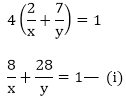Again 4 men and 4 boys can finish the work in 3 days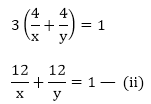Putting 1/x = u and 1/y = v in equation (i) and (ii) we get

8u + 28v = 1

12u + 12v = 1

8u + 28v – 1 = 0 … (iii)

12u + 12v – 1 = 0 … (iv)

By using cross multiplication we have

Now,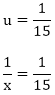x = 15

andy = 60

Hence, one man alone can finish the work in 15 days and one boy alone can finish the work in 60 days.

Question: 7

In a ΔABC, ∠A = x°, ∠B = (3x – 2)°, ∠C = y°, Also, ∠C – ∠B = 9°. Find the three angles.

Solution:

Let ∠A = x°, ∠B = (3x – 2)°, ∠C = y° and

∠C – ∠B = 9°

⇒ ∠C = 9° + ∠B

⇒ ∠C = 9° + 3x° − 2°

⇒ ∠C = 7° + 3x°

Substitute ∠C = y° in above equation we get,

y° = 7° + 3x°

∠A + ∠B + ∠C = 180°

⇒ x° + (3x° − 2°) + (7° + 3x°) = 180°

⇒ 7x° + 5° = 180°

⇒ 7x° = 175°

⇒ x° = 25°

∠A = x° = 25°

∠B = (3x – 2)° = 73°

∠C = (7 + 3x)° = 82°

Hence, ∠A = 25°, ∠B = 73°, ∠C = 82°

Question: 8

In a cyclic quadrilateral ABCD, ∠A = (2x + 4)°, ∠B = (y + 3)°, ∠C = (2y + 10)°, ∠D = (4x – 5)°. Find the four angles.

Solution:

We know that the sum of the opposite angles of cyclic quadrilateral is 180°. In the cyclic quadrilateral ABCD, angles A and C and angles B and D pairs of opposite angles

Therefore ∠A + ∠C = 180° and ∠B + ∠D = 180°

Taking ∠A + ∠C = 180°

By substituting ∠A = (2x + 4)° and ∠C = (2y + 10)° we get

2x + 4 + 2y + 10 = 180°

2x + 2y + 14 = 180°

2x + 2y = 180° - 14°

2x + 2y = 166 ….(i)

Taking  ∠B + ∠D = 180°

By substituting ∠B = (y + 3)° and ∠D = (4x – 5)° we get

y + 3 + 4x – 5 = 180°

4x + y – 5 + 3 = 180°

4x + y – 2 = 180°

4x + y = 180° + 2°

4x + y = 182° ….. (ii)

By multiplying equation (ii) by 2 we get 8x + 2y = 364 …… (iii)

By subtracting equation (iii) from (i) we get

- 6x = - 198

x = -198/-6

x = 33°

By substituting x = 33° in equation (ii) we get

4x + y = 182

132 + y = 182

y = 182 – 132

y = 50

The angles of a cyclic quadrilateral are

∠A = 2x + 4

= 66 + 4

= 70°

∠B = y + 3

= 50 + 3

= 53°

∠C = 2y + 10

= 100 + 10

= 110°

∠D = 4x – 5

= 132 – 5

= 127°

Hence, the angles of cyclic quadrilateral ABCD are ∠A = 70°, ∠B = 53°, ∠C = 110°, ∠D = 127°

Question: 9

In a ΔABC, ∠A = x°, ∠B = 3x°, ∠C = y°. If 3y – 5x = 30, prove that the triangle is right angled.

Solution:

We have to prove that the triangle is right angled.

Given ∠A = x°, ∠B = 3x° and ∠C = y°

Sum of three angles in triangle are ∠A + ∠B + ∠C = 180°

∠A + ∠B + ∠C = 180°

x + 3x + y = 180°

4x + y = 180 — (i)

By solving 4x + y = 180 with 3y – 5x = 30 …..(ii) we get,

Multiplying equation (i) by 3 we get

12x + 36y = 540 ….(iii)

Subtracting equation (ii) from (iii)

17x = 510

x = 510/17

x = 30°

Substituting x = 30° in equation (i) we get

4x + y = 180

120 + y = 180

y = 180 – 120

y = 60°

Angles ∠A, ∠B and ∠C are

∠A = x°

= 30°

∠B = 3x°

= 90°

∠C = y°

= 60°

A right angled triangle is a triangle in which one side should has a right angle that is 90° in it. Hence, ∠B = 90°. The triangle ABC is right angled.

Question: 10

The car hire charges in a city comprise of a fixed charges together with the charge for the distance covered. For a journey of 12 km, the charge paid is Rs. 89 and for a journey of 20 km, the charge paid is Rs. 145. What will a person have to pay for travelling a distance of 30 km?

Solution:

Let the fixed charges of car be Rs. x per km and the running charges be Rs. y km/hr

According to the given condition we have

x + 12y = 89 …. (i)

x + 20y = 145 …. (ii)

By subtracting (i) from (ii) we get

- 8y = - 56

y = – 56/- 8

y = 7

Putting y = 7 in equation (i) we get

x + 12y = 89

x + 84 = 89

x = 89 – 84

x = 5

Therefore, total charges for travelling distance of 30 km

= x + 30y

= 5 + 210

= Rs. 215

Hence, A person have to pay Rs. 215 for travelling a distance of 30 km.

Question: 11

A part of monthly hostel charges in a college are fixed and the remaining depend on the number of days one has taken food in the mess. When a student A takes food for 20 days, he has to pay Rs. 1000 as hostel charges whereas a student B, who takes food for 26 days, pays Rs. 1180 as hostel charges. Find the fixed charge and the cost of food per day.

Solution:

Let the fixed charges of hostel be Rs. x and the cost of food charges be Rs. y per day

According to the given condition we have,

x + 20y = 1000 — (i)

x + 26y = 1180 — (ii)

Subtracting equation (ii) from equation (i) we get

- 6y = - 180

y = – 180/- 6

y = 30

Putting y =30 in equation (i) we get

x + 20y = 1000

x + 600 = 1000

x = 1000 – 600

x = 400

Hence, the fixed charges of hostel is Rs. 400

The cost of food per day is Rs. 30

Question: 12

Half the perimeter of a garden, whose length is 4 more than its width is 36 m. Find the dimension of the garden.

Solution:

Let perimeter of rectangular garden will be 2(l + b). Then half the perimeter of a garden will be 36 m

1/2 x 2(l + b) = 36

l + b = 36 …. (i)

When the length is four more than its width then (b + 4)

Substituting l = b + 4 in equation (i) we get

l + b = 36

b + 4 + b = 36

2b = 36 – 4

2b = 32

b = 32/2

b = 16

Putting b = 16 in equation (i) we get

(l + b) = 36

l + 16 = 36

l = 36 -16

l = 20

Hence, the dimension of rectangular garden are width = 16 m and length = 20 m.

Question: 13

The larger of two supplementary angles exceeds the smaller by 18 degrees. Find them.

Solution:

We know that the sum of supplementary angles will be 180o

Let the larger supplementary angle be x.

We know x + y = 180°  ….. (i)

If larger of supplementary angles exceeds the smaller by 18 degrees, then

x = y + 18  ….. (ii)

Substitute x = y + 18 in equation (i), we get

x + y = 180°

y + 18 + y = 180°

2y + 18 = 180°

2y = 180° – 18°

2y = 162°

y = (162/2)°

y = 81°

Put y = 81° in equation (ii), we get

x = y + 18

x = 81 + 18

x = 90°

Hence, the larger supplementary angle is 99°

The smaller supplementary angle is 81°

Question: 14

2 women and 5 men can together finish a piece of embroidery in 4 days, while 3 women and 6 men can finish it in 3 days. Find the time taken by 1 woman alone to finish the embroidery, and that taken by 1 man alone.

Solution:

Q woman alone can finish the work in x days and 1 man alone can finish it in y days. Then,

One woman one day work = 1/x

One man one day work = 1/y

2 women’s one day work = 2/x

5 men’s one day work = 5/y

Since 2 women and 5 men can finish the work in 4 days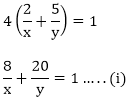3 women and 6 men can finish the work in 3 days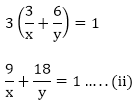Putting 1/x = u and 1/y = v in equation (i) and (ii) we get

8u + 20v - 1 = 0  ….. (iii)

9u + 18v - 1 = 0  ….. (iv)

By using cross multiplication we have

Now,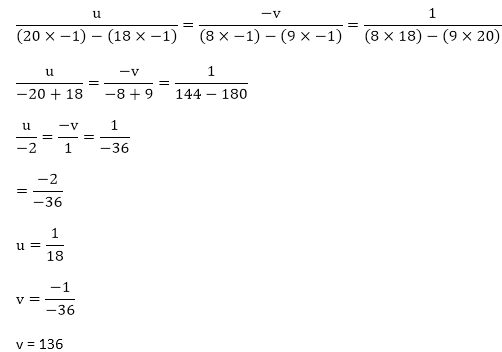v = 136

Now,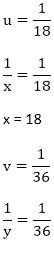Hence, the time taken by 1 woman alone to finish the embroidery is 18 days.

The time taken by 1 man alone to finish the embroidery is 36 days

Question: 15

Meena went to a bank to withdraw Rs. 2000. Shw asked the cashier to give her Rs. 50 and Rs. 100 notes only. Meena got 25 notes in all. Find how many notes Rs. 50 and Rs. 100 she received.

Solution:

Let x and y be the number of notes of Rs. 50 and Rs. 100 respectively

If Meena ask for Rs. 50 and Rs. 100 notes only, then the equation will be,

50x + 100y = 2000

Divide both sides by 50 then we get,

x + 2y = 40 …. (i)

If Meena got 25 notes in all then the equation will be,

x + y = 25 …. (ii)

By subtracting the equation (ii) from (i) we get

y = 15

Substituting y = 15 in equation (ii), we get

x + y = 25

x + 15 = 25

x = 25 – 15

x = 10

Therefore x = 10 and y = 15

Hence, Meena has 10 notes of Rs. 50 and 15 notes of Rs. 100

Question: 16

A wizard having powers of mystic in candations and magical medicines seeing a cock fight going on, spoke privately to both the owners of cocks. To one he said; if your bird wins, then you give me your stake-money, but if you do not win, I shall give you two third of that. Going to the other, he promised in the same way to give three fourth. From both of them his gain would be only 12 gold coins. Find the stake of money each of the cock-owners have.

Solution:

Let the strike money of first cock-owner be Rs. x and of second cock-owner be Rs. y respectively.

Then we have,4x – 3y = 48 …. (i)

For second cock-owner according to given condition we have,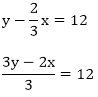3y – 2x = 36   …. (ii)

By subtracting (ii) from (i), we have

2x = 84

x = 84/2 = 42

Putting x = 42 in equation (ii) we get,

3y – 2x = 36

3y – 84 = 36

3y = 36 + 84

3y = 120

y = 120/3 = 40

Hence the stake of money of first cock-owner is Rs. 42 and of second cock-owner is Rs. 40.

Question: 17

The students of a class are made to stand in rows. If 3 students are extra in a row, there would be 1 row less. If 3 students are less in arrow there would be 2 rows more. Find the number of students in the class.

Solution:

Let the number of students be x and the number of rows be y. Then,

Number of students in each row = x/y

Where three students is extra in each row, there are one row less that is when each row has (xy + 3) (x/y + 3) students the number of rows is (y - 1)

Total number of students = number of rows x number of student in each row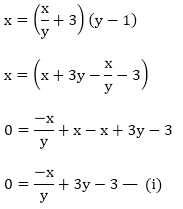If three students are less in each row then there are 2 rows more that is when each row has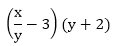Therefore, total number of students = number of rows x number of students in each rowPutting x/y = u in (i) and (ii) we get

- u + 3y – 3 = 0 … (iii)

2u – 3y – 6 = 0 … (iv)

Adding (iii) and (iv) we get

u – 9 = 0

u = 9

Putting u = 9 in equation (iii) we get

- u + 3y – 3 = 0

- 9 + 3y – 3 = 0

3y – 12 = 0

3y = 12

y = 12/3

y = 4

Now,

x/y = 9

x/4 = 9

x = 36

Hence, the number of students in the class is 36.

Question: 18

One says, “Give me hundred, friend! I shall then become twice as rich as you”. The other replies, “If you give me ten, I shall be six times as rich as you”. Tell me what is the amount of their respective capital?

Solution:

Let the money with first person be Rs. x and the money with the second person be Rs. y. Then,

(x + 100) = 2(y – 100)

(y + 10) = 6(x – 10)

If first person gives Rs. 100 to second person then the second person will become twice as rich as first person. According to the given condition, we have,

(x + 100) = 2(y – 100)

x + 100 = 2y – 200

x – 2y + 100 + 200 = 0

x – 2y + 300 = 0 — (i)

If second person gives Rs. 10 to first person then the first person will become six times as rich as second person. According to given condition, we have

(y + 10) = 6(x – 10)

y + 10 = 6x – 60

0 = 6x – 60 – y – 10

0 = 6x – y – 70 …. (ii)

Multiplying (ii) by 2 we get,

12x – 2y – 140 = 0 …. (iii)

By subtracting (iii) from (i), we get

- 11x + 440 = 0

- 11x = – 440

x = 440/11 = 40

Putting x = 40 in (i) we get,

x – 2y + 300 = 0

40 – 2y + 300 = 0

-2y + 340 = 0

2y = 340

y = 340/2 = 170

Hence, first person’s capital will be Rs. 40.

Second person’s capital will be Rs. 170.
```### Course Features

• 728 Video Lectures
• Revision Notes
• Previous Year Papers
• Mind Map
• Study Planner
• NCERT Solutions
• Discussion Forum
• Test paper with Video Solution